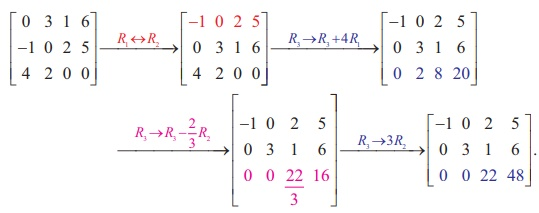Home | | Maths 12th Std | Row Echelon form

# Row Echelon form

Using the row elementary operations, we can transform a given non-zero matrix to a simplified form called a Row-echelon form.

Row-Echelon form

Using the row elementary operations, we can transform a given non-zero matrix to a simplified form called a Row-echelon form. In a row-echelon form, we may have rows all of whose entries are zero. Such rows are called zero rows. A non-zero row is one in which at least one of the entries is not zero. For instance, in the matrix,,

R1 and R2 are non-zero rows and R3 is a zero row

### Definition 1.5

A non-zero matrix E is said to be in a row-echelon form if:

i.                     All zero rows of E occur below every non-zero row of E.

ii.                     The first non-zero element in any row i of E occurs in the jth column of E , then all other entries in the jth column of E below the first non-zero element of row i are zeros.

iii.                     The first non-zero entry in the ith row of E lies to the left of the first non-zero entry in (i +1)th row of E .

Note

A non-zero matrix is in a row-echelon form if all zero rows occur as bottom rows of the matrix, and if the first non-zero element in any lower row occurs to the right of the first non- zero entry in the higher row.

The following matrices are in row-echelon form:Consider the matrix in (i). Go up row by row from the last row of the matrix. The third row is a zero row. The first non-zero entry in the second row occurs in the third column and it lies to the right of the first non-zero entry in the first row which occurs in the second column. So the matrix is in row- echelon form.

Consider the matrix in (ii). Go up row by row from the last row of the matrix. All the rows are non-zero rows. The first non-zero entry in the third row occurs in the fourth column and it occurs to the right of the first non-zero entry in the second row which occurs in the third column. The first non-zero entry in the second row occurs in the third column and it occurs to the right of the first non-zero entry in the first row which occurs in the first column. So the matrix is in row-echelon form.

The following matrices are not in row-echelon form:Consider the matrix in (i). In this matrix, the first non-zero entry in the third row occurs in the second column and it is on the left of the first non-zero entry in the second row which occurs in the third column. So the matrix is not in row-echelon form.

Consider the matrix in (ii). In this matrix, the first non-zero entry in the second row occurs in the first column and it is on the left of the first non-zero entry in the first row which occurs in the second column. So the matrix is not in row-echelon form.

### Method to reduce a matrix  [aij]m Ă—nto a row-echelon form.

Step 1

Inspect the first row. If the first row is a zero row, then the row is interchanged with a non-zero row below the first row. If a11 is not equal to 0, then go to step 2. Otherwise, interchange the first row R1 with any other row below the first row which has a non-zero element in the first column; if no row below the first row has non-zero entry in the first column, then consider a12 . If a12 is not equal to 0, then go to step 2. Otherwise, interchange the first row R1 with any other row below the first row which has a non-zero element in the second column; if no row below the first row has non-zero entry in the second column, then consider a13. Proceed in the same way till we get a non-zero entry in the first row. This is called pivoting and the first non-zero element in the first row is called the pivot of the first row.

Step 2

Use the first row and elementary row operations to transform all elements under the pivot to become zeros.

Step 3

Consider the next row as first row and perform steps 1 and 2 with the rows below this row only.

Repeat the step until all rows are exhausted.

### Example 1.13

Reduce the matrixto a row-echelon form.

### SolutionNoteThis is also a row-echelon form of the given matrix.

So, a row-echelon form of a matrix is not necessarily unique.

Example 1.14

Reduce the matrixto a row-echelon form.

SolutionTags : Definition, Theorem, Formulas, Solved Example Problems | Elementary Transformations of a Matrix , 12th Mathematics : UNIT 1 : Applications of Matrices and Determinants
Study Material, Lecturing Notes, Assignment, Reference, Wiki description explanation, brief detail
12th Mathematics : UNIT 1 : Applications of Matrices and Determinants : Row Echelon form | Definition, Theorem, Formulas, Solved Example Problems | Elementary Transformations of a Matrix